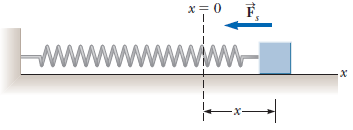# Problem: The figure shows a light extended spring exerting a force Fs to the left on a block.(i) Does the block exert a force on the spring? Select all that apply.a) Its magnitude is equal to Fs.b) Yes, to the right.c) Its magnitude is larger than Fs.d) Yes, to the left.e) No, it does not.f) Its magnitude is smaller than Fs.(ii) Does the spring exert a force on the wall? Select all that apply.a) Its magnitude is smaller than Fs.b) Yes, to the right.c) Its magnitude is equal to Fs.d) Its magnitude is larger than Fs.e) No, it does not.f) Yes, to the left.

###### FREE Expert Solution

From Newton's third law, Action and Reaction are equal but opposite.

(i)

The block pulls the spring with a force equal to Fs directed to the right.

79% (23 ratings)###### Problem Details

The figure shows a light extended spring exerting a force Fs to the left on a block.(i) Does the block exert a force on the spring? Select all that apply.

a) Its magnitude is equal to Fs.

b) Yes, to the right.

c) Its magnitude is larger than Fs.

d) Yes, to the left.

e) No, it does not.

f) Its magnitude is smaller than Fs.

(ii) Does the spring exert a force on the wall? Select all that apply.

a) Its magnitude is smaller than Fs.

b) Yes, to the right.

c) Its magnitude is equal to Fs.

d) Its magnitude is larger than Fs.

e) No, it does not.

f) Yes, to the left.